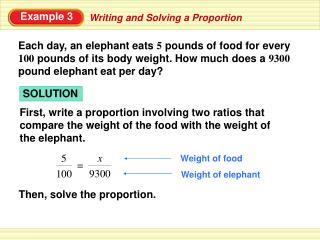DownloadDownload PresentationExample 3

# Example 3

Télécharger la présentation## Example 3

- - - - - - - - - - - - - - - - - - - - - - - - - - - E N D - - - - - - - - - - - - - - - - - - - - - - - - - - -
##### Presentation Transcript

1. Example 3 SOLUTION Weight of food x 5 = First, write a proportion involving two ratios that compare the weight of the food with the weight of the elephant. 9300 100 Weight of elephant Writing and Solving a Proportion Each day, an elephant eats 5 pounds of food for every 100 pounds of its body weight. How much does a 9300pound elephant eat per day? Then, solve the proportion.

2. Example 3 x = 9300 • Multiply each side by 9300. 9300 46,500 = x Simplify. 100 465 x Divide. = 5 100 ANSWER A 9300 pound elephant eats about 465 pounds of food per day. 9300 • Writing and Solving a Proportion

3. Checkpoint about625lb ANSWER 9. Each day, an elephant eats 5 pounds of food forevery 100 pounds of its body weight. How much does a 12,500 pound elephant eat per day?